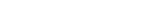MathExamle.com# Equation

Select from the list a generator that creates equations

Creates linear equations, the answers are always positive and integer

4 Variants 3 Levels
 x÷2=402 x÷91=7 x÷149=5 864÷x=36 651÷x=31 88×x=880
Variant: 2 Level: Difficult

Creates linear equations, the answers are integers and can be negative

4 Variants 3 Levels
 -x×61=-610 -x÷(-4)=97 615÷(-x)=41 -42×x=-420 -x×(-2)=-700 -760÷x=76
Variant: 2 Level: Difficult

Creates system of equations, the answers are always integers and positive

3 Variants 3 Levels
 28y÷7x=10 2y×11x=880
 90y÷10x=30 x×12y=360
Variant: 2 Level: Easy